Courses

# Newton's Universal Law of Gravitation and Gravitational Force Class 9 Notes | EduRev

## Class 9 : Newton's Universal Law of Gravitation and Gravitational Force Class 9 Notes | EduRev

The document Newton's Universal Law of Gravitation and Gravitational Force Class 9 Notes | EduRev is a part of the Class 9 Course Science Class 9.
All you need of Class 9 at this link: Class 9

Gravitation

• The paths followed by the planets around the sun and by the satellites around the planets are known as orbits.
• According to Newton's first law of motion, these planets and satellites can move in circular orbits only if some force is acting on them. The mystery of the motion of all these heavenly bodies (i.e. planets and satellites) in nearly circular orbits was solved by Isaac Newton when he observed that an apple fall from a tree towards the earth.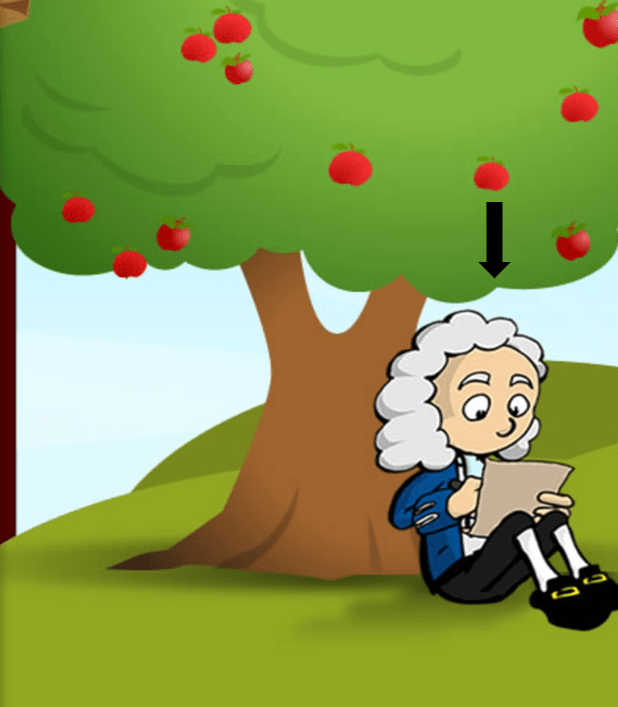• Therefore, he proposed that all particles or objects in the universe attract each other in the same manner as the earth attracted the apple.
• "The force of attraction between any two particles in the universe is called gravitation or gravitation force".

➢ Centripetal Force

• The force acting towards the centre is called centripetal force.
Example: Consider a girl whirling a ball along a circular path. If the girl releases the ball at some point, the ball flies off along the tangent, at that point on the circular path. Let us discuss this observation carefully.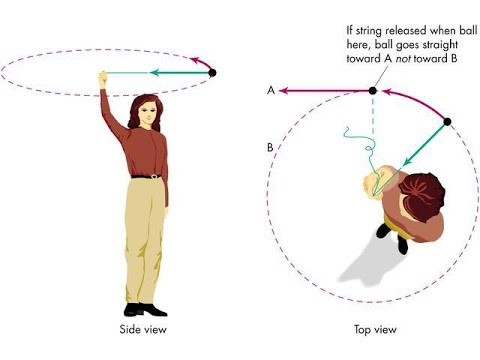A ball describing a circular path with a velocity of constant magnitude

• Before the release of thread, the ball was moving with a certain uniform speed and changed its direction at every point. Because of the change in direction, it moved with a variable velocity and has some definite acceleration.
• The force that causes this acceleration and makes the ball move along the circular path, acts towards the centre, i.e., towards the hand of the girl. This force is called the centripetal force.
•  When the thread is released, the ball does not experience the centripetal force and flies off along a straight line. This straight line is always tangent to the circular path.

➢ Newton's Universal Law of Gravitation

• Newton's universal law of gravitation states that "Every particle in the universe attracts every other particle with of force which is directly proportional to the product of two masses and inversely proportional to the square of the distance between them ".
• The direction of the force is along the line joining the two masses.
• If m1 and m2 are the masses of two bodies separated by a distance d and F is the force of attraction between them, then we get: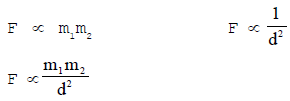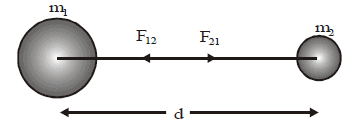• or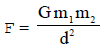where G is a constant of proportionality and known as the constant of universal gravitation and is equal to: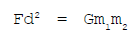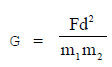• If m1 = m2 = 1kg and d = 1m, then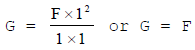• i.e. Universal gravitational constant is the force of attraction (in newton) between two bodies of mass 1 kg each lying 1 metre distance apart.
• Unit of gravitational constant: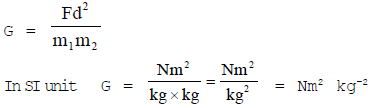In CGS unit = dyn cm2 g-2.

➢ Characteristics of Gravitational Force

• Gravitational force between two bodies or object does not need any contact between them. It means, gravitational force is action at a distance.
• Gravitational force between two bodies varies inversely proportional to the square of the distance between them. Hence, gravitational force is an inverse square force.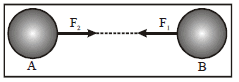• The gravitational forces between two bodies or objects form an action-reaction pair. If object A attract object B with a force F1 and the object B attracts object A with a force F2, then F1 = F2.

Do You Know?

• The value of G = 6.67 × 10-11 N m2 kg-2 or 6.67 × 10-8 dyn cm2 g-2.
• The value of G is same throughout the universe and hence G is known as universal gravitational constant.
• Value of G was determined in laboratory by Sir Henry Cavendish.
• Since the value of G is very small, so the gravitational force is a very weak force.
• The gravitational force between a body on the earth is called gravity.

➢ Importance of Universal Law of Gravitation

The Universal law of gravitation given by Newton has explained successfully several phenomena such as:

• The gravitational force of attraction of the Earth is responsible for binding all terrestrial objects on the Earth.
• The gravitational force of the Earth is responsible for holding the atmosphere around the Earth.
• The gravitational force of the Earth is also responsible for the rainfall and snowfall on the Earth.
• The flow of water in rivers is also due to gravitational force of the Earth on water.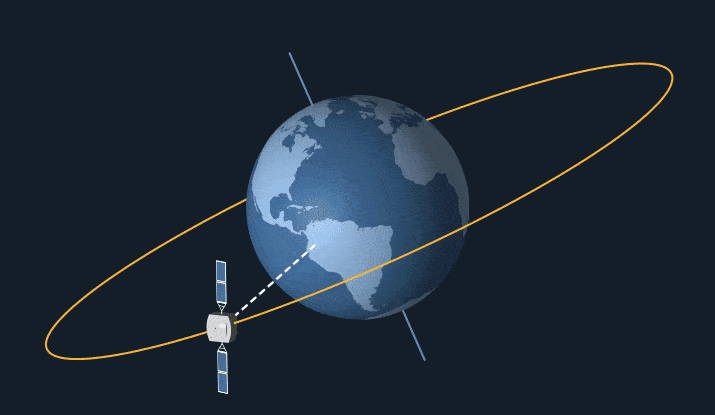A Satellite Revolving Around the Earth
• The moon revolves around the Earth on account of gravitational pull of the Earth on the Moon. Even all artificial satellites revolve around the Earth on account of gravitational pull of the Earth on the satellites.
• The predictions about solar and lunar eclipses made on the basis of this law always come out to be true. The gravitational force plays an important role in nature.
• All the planets revolve around the sun due to the gravitational force between the sun and the planets. The force required by a planet to move around the sun in circular path (known as centripetal force) is provided by the gravitational force of attraction between the planet and the sun. Thus, gravitational force is responsible for the existence of the solar system.
• Tides in oceans are formed due to the gravitational force between the moon and the water in oceans.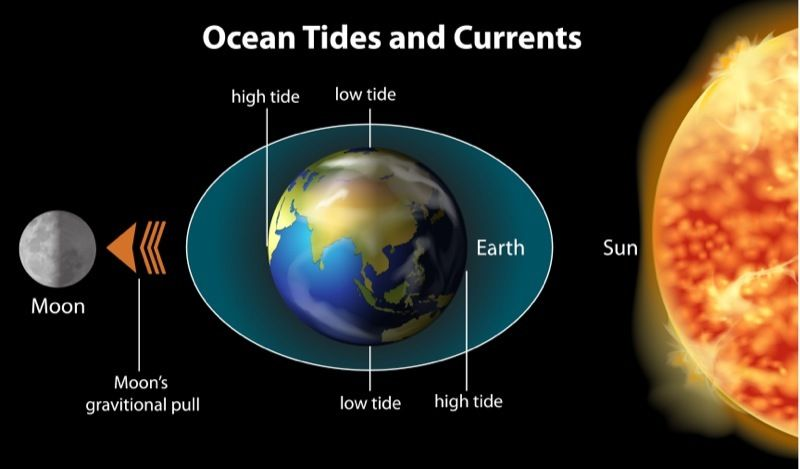• Gravitational force between a planet and its satellite (i.e., moon) decides whether a planet has a moon or not. Since the gravitational force of the planets like Mercury and Venus is very small, therefore, these planets do not have any satellite or moon.
• We stay on the earth due to the gravitational force between the earth and us.

➢ Gravitational Force Between Light Objects and Heavy Objects

The formula applied for calculating gravitational force between light objects and heavy objects is the same, i.e.,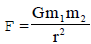Let us take three cases:

(i) When two bodies of mass 1 kg each are 1 metre apart.

Sol.
m1= m2 = 1 kg, r = 1 m
Taking G = 6·67 × 10-11 Nm2/kg2, we obtain gravitational force of attraction,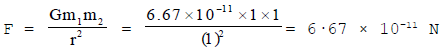which is extremely small. Hence, we conclude that though every pair of two objects exert gravitational pull on each other, yet they cannot move towards each other because this gravitational pull is too weak.

(ii) When a body of mass 1 kg is held on the surface of Earth.

Sol.
Here, m1 = 1 kg
m2 = mass of Earth = 6 × 1024 kg
r = distance of body from centre of Earth = radius of Earth = 6400 km = 6.4 × 103km = 6·4 × 106 m

Gravitational force of attraction between the body and Earth,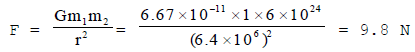It means that the Earth exerts a gravitational force of 9·8 N on a body of mass one kg. This force is much larger compared to the force when both the bodies are lighter. That is why when a body is dropped from a height, it falls to the Earth.

(iii) When both the body are heavy.
Sol.
Let us calculate gravitational force of attraction between Earth and the Moon.
Mass of Earth, m1 = 6 × 1024 kg
Mass of Moon, m2 = 7.4 × 1022 kg
Distance between Earth and Moon, r = 3.84 × 105 km = 3.84 × 108 m
Gravitational constant, G = 6·67 × 10-11 Nm2/kg2
The gravitational force between Earth and Moon,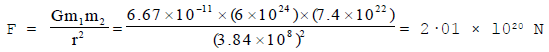which is really large. It is this large gravitational force exerted by Earth on Moon, which makes the Moon revolve around the Earth.

Offer running on EduRev: Apply code STAYHOME200 to get INR 200 off on our premium plan EduRev Infinity!

## Science Class 9

95 videos|223 docs|135 tests

,

,

,

,

,

,

,

,

,

,

,

,

,

,

,

,

,

,

,

,

,

;